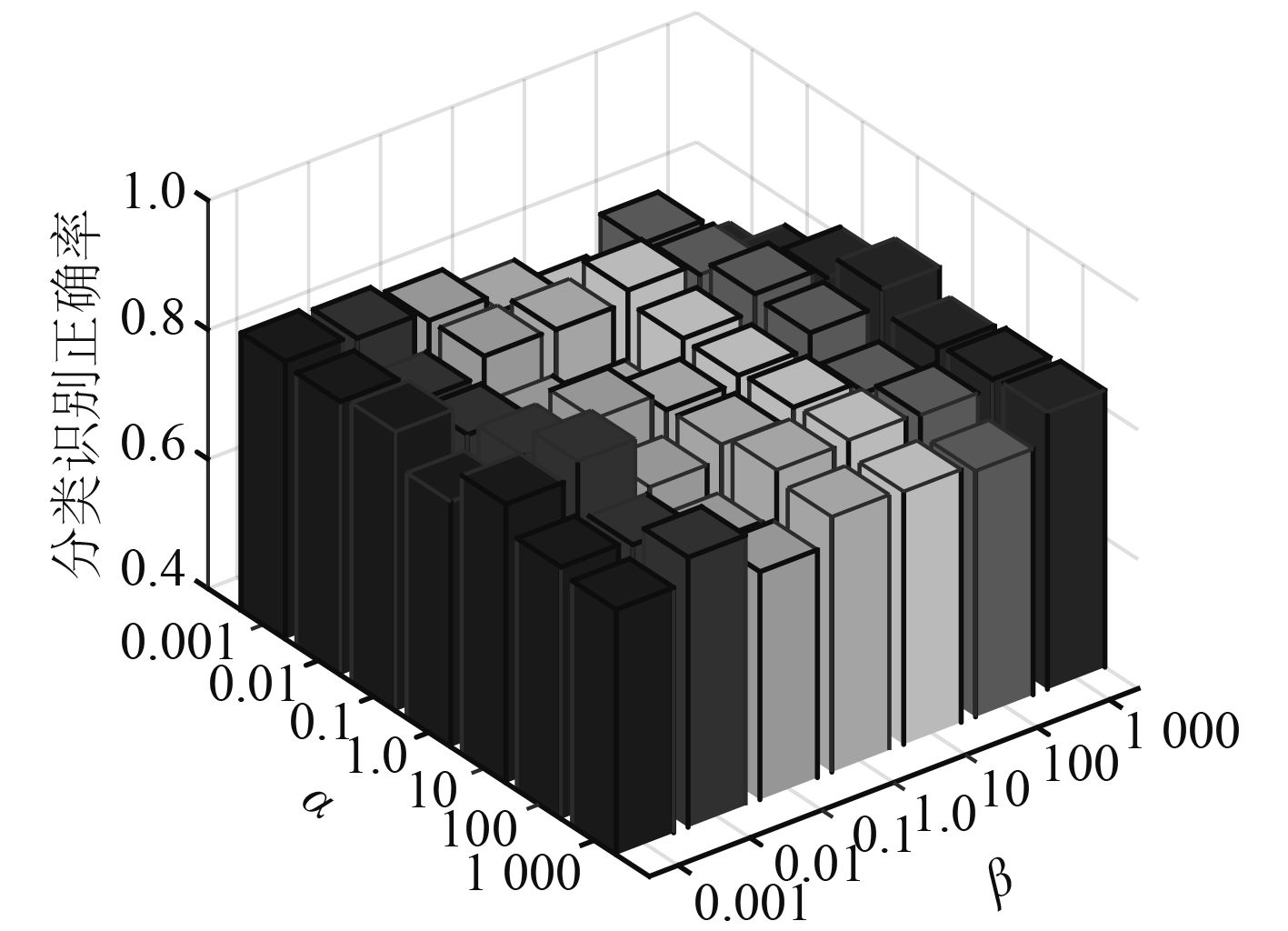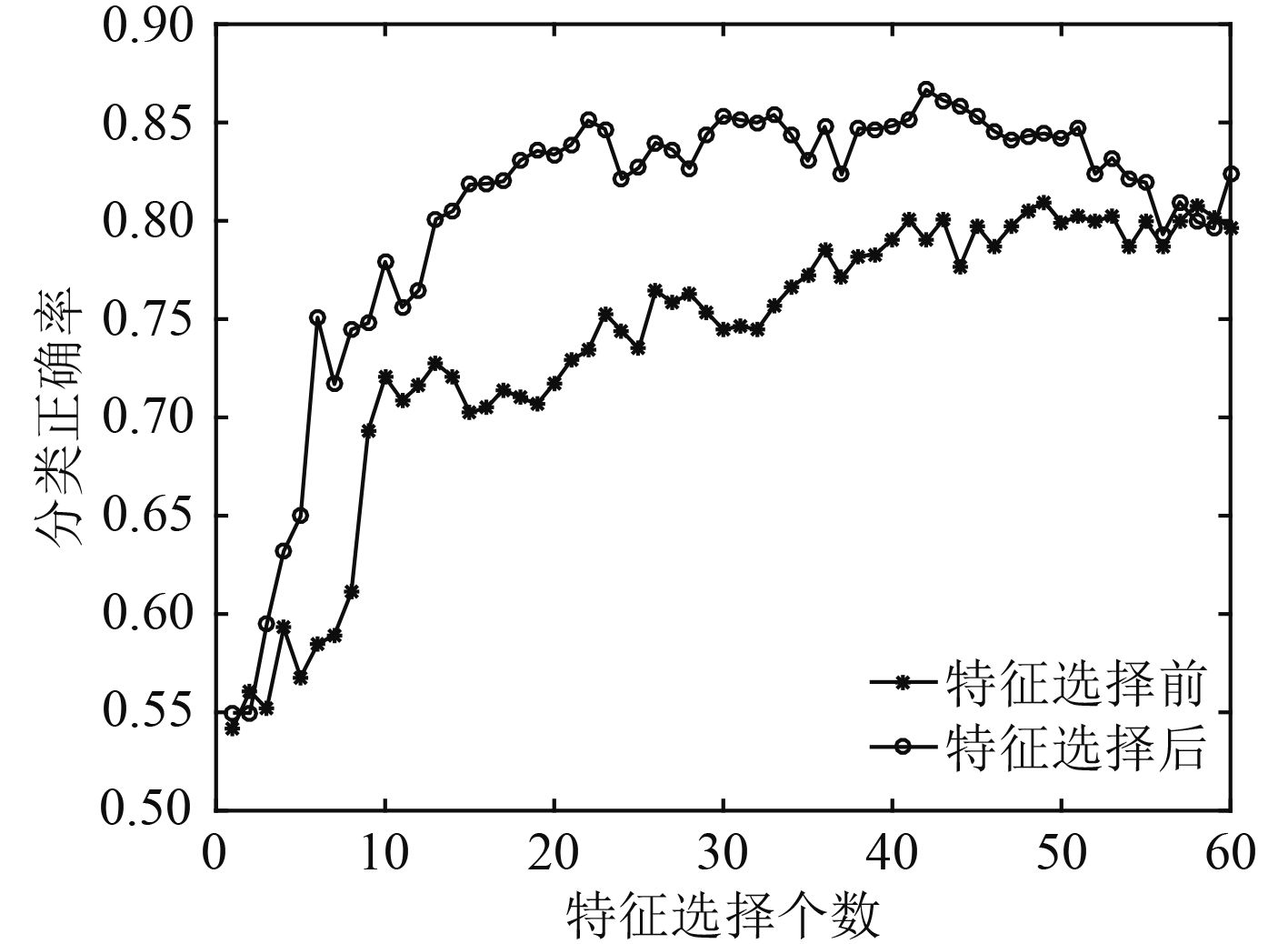﻿ 用于水下目标识别的无监督特征选择算法
 舰船科学技术2017, Vol. 39Issue (12): 91-94PDF

Unsupervised feature selection algorithm for underwater target recognition
YANG Hong-hui, LI Jiang-tao, SHEN Sheng, YAO Xiao-hui
School of Marine Science and Technology, Northwestern Polytechnical University, Xi'an, 710072, China
Abstract: With the development of society, marine space becomes more and more important to human beings, and the demand for new automatic identification system for underwater targets is becoming more and more urgent. In the construction of the underwater target automatic identification system, the extracted features contain many redundant, irrelevant and noise features, which affect the efficiency of the system and reduce the accuracy of classification and recognition. To this end, we proposed a new feature selection algorithm for underwater target recognition-Unsupervised Feature Selection Algorithm Based on Graph Learning (UFSGL). The algorithm framework is optimized the transformation matrix and graph learning at the same time, and use the regularization method to optimize the smoothness of the weighted edge. Using the sonar dataset of UCI database to verify the performance of the algorithm, the results show that UFSGL algorithm can effectively reduce the number of features in feature subsets and improve the accuracy of classification recognition to a certain extent.
Key words: unsupervised     feature selection     graph learning     underwater target recognition
0 引　言

1 相关术语 1.1 范数

 ${\left\| A \right\|_{r,s}} = {\left( {\sum\limits_{i = 1}^e {{{\left( {\sum\limits_{j = 1}^f {{{\left| {{A_{ij}}} \right|}^r}} } \right)}^{s/r}}} } \right)^{1/s}}\text{。}$ (1)
1.2 局部保留投影

 $\arg \mathop {\min }\limits_W \sum\limits_{i,j = 1}^n {{{\left\| {{W^{\rm T}}{x_i} - {W^{\rm T}}{x_j}} \right\|}^2}{S_{ij}}}\text{。}$ (2)

LPP的基本思想是，寻找转换矩阵W，将高维数据X转换到低维矩阵XW，以用于最大化地保持X的局部结构的连接，最小化式（2）以确保当xixj相邻时， ${W^{\rm T}}{x_i}$ ${W^{\rm T}}{x_j}$ 也相邻。

2 自适应图学习无监督特征选择方法

2.1 图的构建

 ${{S}_{ij}}= {e^{ - \displaystyle\frac{{{{\left\| {{x_i} - {x_j}} \right\|}^2}}}{{2{\delta ^2}}}}}\text{。}$ (3)

 ${L} ={D} - {S}\text{。}$ (4)
2.2 目标函数

 $\begin{array}{l}\mathop {\min }\limits_{S,W} \sum\limits_{i,j = 1}^n {\left( {\left\| {{W^{\rm T}}{x_i} \!-\! {W^{\rm T}}{x_j}} \right\|_2^2{S_{ij}}} \right)} \!+\! \alpha \sum\limits_{i,j = 1}^n {S_{ij}^{}} \!+\! \beta {\left\| W \right\|_{2,1}}\\\begin{array}{*{20}{c}}{\rm s.t.} & {0 \leqslant {S_{ij}} \leqslant 1,{W^{\rm T}}{S_t}W = I}\text{。}\end{array}\end{array}$ (5)

3 目标函数的优化

 ${U_{ii}} = \frac{1}{{2{{\left\| {{w_i}} \right\|}_2}}}\text{。}$ (6)

 $\begin{array}{l}\mathop {\min }\limits_{S,W,U} tr\left( {{{W}^{\rm T}}{X}{{L}_S}{{X}^{\rm T}}W} \right) + \beta tr\left( {{{W}^{\rm T}}{UW}} \right)\text{，}\\\begin{array}{*{20}{c}}{\rm s.t.}&{{{W}^{\rm T}}{{S}_t}{W} = I}\text{。}\end{array}\end{array}$ (7)

 $\begin{array}{l}\mathop {\min }\limits_{W,U} tr\left( {{{W}^{\rm T}}\left( {{X}{{L}_S}{{X}^{\rm T}} + \beta {U}} \right){W}} \right),\\\begin{array}{*{20}{c}}{\rm s.t.}&{{{W}^{\rm T}}{{S}_t}{W} = I}{\text{。}}\end{array}\end{array}$ (8)

 ${L}({S_{ij}}) =\!\!\! \sum\limits_{i,j = 1}^n {\left( {\left\| {{{W}^{\rm T}}{x_i} \!-\! {{W}^{\rm T}}{x_j}} \right\|_2^2{{S}_{ij}}} \right)} \!\!+\!\! \alpha \sum\limits_{i,j = 1}^n {{{S}_{ij}}} + {\psi _{ij}}{{S}_{ij}}\text{。}$ (9)

 $\frac{{\partial {L}({{bm S}_{ij}})}}{{\partial {S_{ij}}}} = \left\| {{{W}^{\rm T}}{x_i} - {{W}^{\rm T}}{x_j}} \right\|_2^2 + \alpha + {\varPsi _{ij}}\text{。}$ (10)

 $\left( {\left\| {{{W}^{\rm T}}{x_i} - {{W}^{\rm T}}{x_j}} \right\|_2^2 + \alpha } \right){{S}_{ij}} = 0\text{。}$ (11)

 ${{\hat {S}}_{ij}} = \frac{{\alpha {{S}_{ij}}}}{{\left\| {{{W}^{\rm T}}{x_i} - {{W}^{\rm T}}{x_j}} \right\|_2^2}}\text{。}$ (12)表 1 UFSGL算法流程 Tab.1 The processing flow of UFSGL
4 实验

4.1 数据介绍表 2 数据集说明 Tab.2 Data specification
4.2 参数设置图 1 $\alpha ,\beta$ 参数对分类识别正确率的影响 Fig. 1 Influence of parameters $\alpha ,\beta$ on the classification accuracy of sonar data

4.3 SVM分类实验及结果图 2 特征选择前后SVM分类识别正确率 Fig. 2 The SVM classification accuracy before and after the feature selection

5 结　语

  ROBNIKŠIKONJA M, KONONENKO I. Theoretical and Empirical Analysis of ReliefF and RReliefF[J]. Machine Learning, 2003, 53 (1-2): 23–69.  DUDA R O, HART P E, STORK D G. Pattern classification. 2nd ed.[J]. Pattern Analysis & Applications, 2001, 1 (1): 142–143.  PENG H, LONG F, DING C. Feature selection based on mutual information criteria of max-dependency, max-relevance, and min-redundancy[J]. IEEE Transactions on Pattern Analysis & Machine Intelligence, 2005, 27 (8): 1226.  MITRA P, MURTHY C A, PAL S K. Unsupervised Feature Selection Using Feature Similarity[J]. Pattern Analysis & Machine Intelligence IEEE Transactions on, 2002, 24 (3): 301–312.  DY J G, BRODLEY C E. Feature Selection for Unsupervised Learning[J]. Journal of Machine Learning Research, 2004, 5 (4): 845–889.  申昇, 杨宏晖, 袁帅. 用于水声目标识别的互信息无监督特征选择[J]. 声学技术, 2013, 32 (6): 30–33. SHEN S, YANG HH, YUAN S. Unsupervised Feature Selection Approach Based on Mutual Information for Underwater Acoustic Target Classification[J]. Technical Acoustics, 2013, 32 (6): 30–33.  HE X, CAI D, NIYOGI P. Laplacian score for feature selection[C]//International Conference on Neural Information Processing Systems. MIT Press, 2005: 507–514.  ZHAO Z, LIU H. Spectral feature selection for supervised and unsupervised learning[C]//Machine Learning, Proceedings of the Twenty-Fourth International Conference. DBLP, 2007: 1151–1157.  CAI D, ZHANG C, HE X. Unsupervised feature selection for multi-cluster data[C]//ACM SIGKDD International Conference on Knowledge Discovery and Data Mining. ACM, 2010: 333–342.  HOU C, NIE F, LI X, et al. Joint embedding learning and sparse regression: a framework for unsupervised feature selection[J]. IEEE Transactions on Cybernetics, 2014, 44 (6): 793. DOI: 10.1109/TCYB.2013.2272642  X NIYOGI, " Locality preserving projections,” in Neural information processing systems. MIT, 2004.